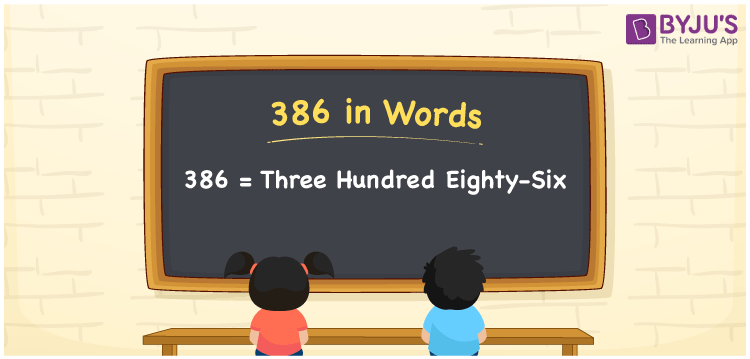# 386 in Words

The number 386 in words is “three hundred eighty-six”. The numeral 386 has three-digits and it is a natural number. Using the place values of the number, we can easily convert the number 386 into words. Also, the number 386 is a cardinal number. For example, the cost of 4 kg oranges is Rs. 386. It can also be written as “The cost of 4 kg oranges is Rs. Three hundred eighty-six”.

 386 in Words: Three Hundred Eighty-six. Three Hundred Eighty-six in Numerical Form: 386.

## 386 in English Words## How to Write 386 in Words?

The place value table for the number 386 is presented below:

 Hundreds Tens Ones 3 8 6

The expanded form of 386 is as follows:

= 3 × Hundred + 8 × Ten + 6 × One

= 3 × 100 + 8 × 10 + 6 × 1

= 300 + 80 + 6

= 386

= Three hundred eighty-six

Hence, 386 in words is three hundred eighty-six.

386 in words – Three hundred eighty-six

Is 386 an odd number? – No

Is 386 an even number? – Yes

Is 386 a perfect square number? – No

Is 386 a perfect cube number? – No

Is 386 a prime number? – No

Is 386 a composite number? – Yes

## Frequently Asked Questions on 386 in Words

Q1

### How to spell 386 in English words?

386 in words is three hundred eighty-six.

Q2

### Simplify 300 + 86, and express it in words.

Simplifying 300 + 86, we get 386. Hence, 386 in words is three hundred eighty-six.

Q3

### Is 386 a composite number?

Yes, 386 is a composite number.